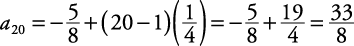## Arithmetic Sequence

An arithmetic sequence is a sequence in which, beginning with the second term, each term is found by adding the same value to the previous term. Its general term is described by

a n = a 1 + ( n –1) d

The number d is called the common difference. It can be found by taking any term in the sequence and subtracting its preceding term.

##### Example 1

Find the common difference in each of the following arithmetic sequences. Then express each sequence in the form a n = a 1 + (n – 1) d and find the twentieth term of the sequence.

1. 1, 5, 9, 13, 17, …

2.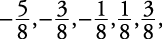1. 1, 5, 9, 13, 17, …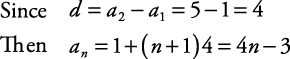Therefore, the twentieth term of the sequence is

a 20 = 1 + (20 – 1)4 = 1 + 76 = 77

2.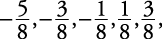Since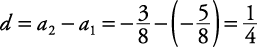ThenTherefore, the twentieth term of the sequence is